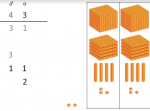Smartick is an advanced online program that teaches kids math and coding in only 15 min. a day

# I want to learn about: Number & Operations In Base Ten

Content about numbers for elementary mathematics. Exercises, tutorials, problems, and teaching resources for elementary aged children. Here you will find posts about numbers to make learning mathematics easier and more fun.

Dec12

## Square Number: Some Tricks and Examples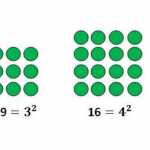In this post, we are going to learn some tricks or strategies for being capable of calculating a square number mentally. What is a square number? A square number or a number raised to the power of 2, is a number multiplied by the same number.  In those cases the multiplication is simple, but if […]

Nov10

## Learn the Signs of the Power: Positive or Negative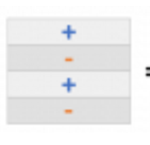In this post, we’re going to decipher an answer’s sign by looking at the sign of a number’s power. To start off, let’s look at an example of what happens when the factor’s signs mingle. As we already know, we multiply the factors normally and later, we add the appropriate sign to the answer. Let’s […]

Oct24

## Powers in Math

Hello! How did your week start off? Are you ready to learn about what powers are today? Yes! You heard correctly, today we are going to focus on powers. Do you know what powers are for? We use powers to simplify multiplication problems that use more than one of the same number. The power of […]

Sep30

## What Are Decimal Numbers?

What are decimal numbers? Decimal numbers are used to represent numbers that are smaller than 1 unit. Decimals are written to the right of the units place separated by a period. That is the say: Hundreds Tens Units; Tenths Hundredths Thousandths In the image that appears below, the first square represents the unit 1. If […]

Jul07

## Units, Tens, Hundreds. Learn How They Are Used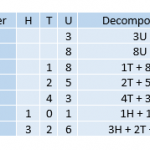In today’s post, we are going to review Units, Tens, Hundreds, and see some examples of how they are used. Units It is the smallest natural number. We are going to represent a unit with a cube: To abbreviate the word unit, we will write U, for example: Tens Let’s look at a larger number […]

Jun29

In this post, we are going to learn what exponents are and how to calculate them. Exponents are used to simplify multiplication problems where the same number is multiplied. For example: 5 x 5 x 5 x 5 We are multiplying the number 5 four times. In order to write it in powers, we first write down […]

Jun10

## Dividing a Decimal by a Whole Number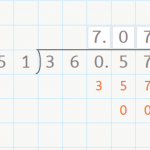Dividing a Decimal by a Whole Number Hello everyone! In this week’s post, we are going to talk about dividing a decimal by a whole number. You already know how to divide a whole number by a whole number by now, right? If not, check out our posts about dividing by a one-digit number here, […]